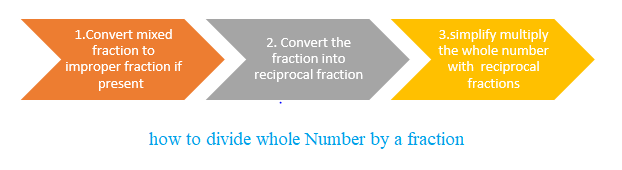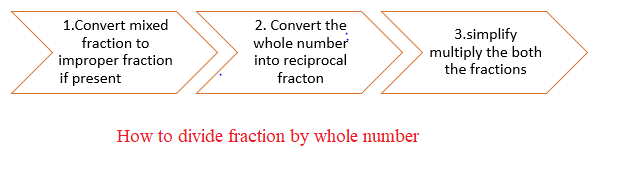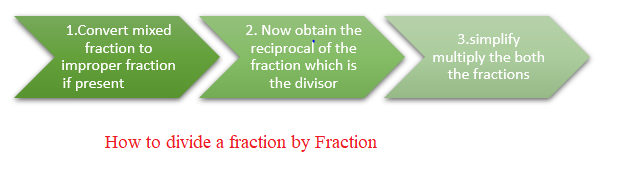# How to Divide fractions | dividing mixed fractions

## Division of Whole Number by a Fraction

Division of Whole Number by a Fraction can be understood by below example
$1 \div \frac {1}{2}$
This can be understood as we have 1 full pizza and we divide into two equal piece. The above division can be understood as how many 1/2 part can be obtained from 1 whole. Obviously it is 2 ,So
$1 \div \frac {1}{2} = 2$
or
$1 \div \times \frac {2}{1} = 2$

The conversion of $\frac {1}{2}$ to $\frac {2}{1}$ is called reciprocal of fraction. $\frac {2}{1}$ is called the reciprocal of fraction $\frac {1}{2}$.
Few examples on reciprocal of fraction
1. $\frac {1}{2}$
Reciprocal Fraction = $\frac {2}{1}$
2. $\frac {2}{3}$
Reciprocal Fraction = $\frac {3}{2}$
3. $\frac {4}{9}$
Reciprocal Fraction = $\frac {9}{4}$
4. 4
Reciprocal Fraction = $\frac {1}{4}$

So steps for Division of Whole Number by a Fraction can be summarized as How to divide whole Number by a fraction
• if we have Mixed Fraction in the division, first convert into Improper Fraction
• Now obtain the reciprocal of the fraction part
• Now We can simplify multiply the whole number with reciprocal fractions .If the results is improper fraction, you can convert into mixed fraction if required.Solved Examples
Question 1. $3 \div \frac {1}{5}$
$3 \div \frac {1}{5}=3 \times \frac {5}{1} =15$

Question 2. $2 \div \frac {4}{5}$
$2 \div \frac {4}{5}=2 \times \frac {5}{4} = \frac {2 \times 5}{4}=\frac {10}{4} = \frac {5}{2} =2 \frac {1}{2}$

Question 3. $2 \div 1\frac {1}{5}$
$2 \div 1\frac {1}{5}= 2 \div \frac {6}{5} = 2 \times \frac {5}{6}=\frac {10}{6} = \frac {5}{3}= 1 \frac {2}{3}$

Question 4. $2 \div \frac {1}{8}$
$2 \div \frac {1}{8} =2 \times \frac {8}{1} =16$

## Division of a Fraction by a Whole Number

Division of a fraction by a whole number is done in the similar manner.
$\frac {1}{8} \div 2 =\frac {1}{8} \times \frac {1}{2} =\frac {1}{16}$

Here are the steps
• if we have mixed fraction, first convert into improper fraction
• Now obtain the reciprocal of the whole number
• Now We can simplify multiply both the fractions .If the results is improper fraction, you can convert into mixed fraction if required.Solved Examples
Question 1. $\frac {1}{5} \div 20$
$\frac {1}{5} \div 20 = \frac {1}{5} \times \frac {1}{20}= \frac {1}{100}$

Question 2. $\frac {2}{5} \div 10$
$\frac {2}{5} \div 10= \frac {2}{5} \times \frac {1}{10}=\frac {2}{50} =\frac {1}{25}$
Question 3. $1\frac {1}{5} \div 3$
$1\frac {1}{5} \div 3= \frac {6}{5} \div 3= \frac {6}{5} \times \frac {1}{3}=\frac {6}{15} =\frac {2}{5}$

### How to divide fraction by a fraction

we have already see the division of fraction by whole number.Let us understand How to divide fraction by a fraction.
$\frac {1}{5} \div \frac {5}{6}= \frac {1}{5} \times \frac {6}{5} = \frac {6}{25}$

Here we can see that dividing fraction is simply acheived by .This same steps applies for how to divide mixed fractions
• if we have mixed fraction, first convert into improper fraction
• Now obtain the reciprocal of the fraction which is the divisor
• Now We can simplify multiply both the fractions .If the results is improper fraction, you can convert into mixed fraction if required.Solved Examples
Question 1. $\frac {3}{4} \div \frac {1}{2}$
$\frac {3}{4} \div \frac {1}{2} =\frac {3}{4} \times \frac {2}{1}= \frac {3 \times 2}{4}= \frac {6}{4}=\frac {3}{1}=1\frac {1}{2}$

Question 2. $\frac {1}{7} \div \frac {4}{9}$
$\frac {1}{7} \div \frac {4}{9}=\frac {1}{7} \times \frac {9}{4} = \frac {9}{28}$

Question 3. $\frac {3}{7} \div 2\frac {1}{5}$
$\frac {3}{7} \div 2\frac {1}{5} =\frac {3}{7} \div \frac {11}{5} = \frac {3}{7} \times \frac {5}{11}=\frac {15}{77}$

Question 4. $2\frac {1}{5} \div 1\frac {1}{2}$
$2\frac {1}{5} \div 1\frac {1}{2} =\frac {11}{5} \div 1\frac {3}{2} =\frac {11}{5} \times \frac {2}{3} = \frac {22}{15} = 1 \frac {7}{15}$
Checkout Dividing Fractions calculator

### Quiz Time

Question 1$3 \div \frac {1}{2}$?
A) $6$
B)$\frac {1}{6}$
C)$\frac {2}{3}$
D)$\frac {3}{2}$
Question 2 $\frac {1}{12} \div 3$?
A)$\frac {1}{48}$
B)$\frac {1}{36}$
C) $\frac {1}{4}$
D)None of these
Question 3 $\frac {1}{2} \div \frac {4}{3}$
A)$\frac {3}{2}$
B)$\frac {2}{3}$
C)$\frac {1}{3}$
D)$1\frac {1}{2}$
Question 4 $\frac {2}{9} \div \frac {1}{9}$
A)$2$
B) $\frac {2}{81}$
C) $\frac {81}{2}$
D) $81$
Question 5 The reciprocal of fraction $1 \frac {2}{3}$?
A)$\frac {2}{5}$
B)$\frac {5}{3}$
C)$\frac {3}{2}$
D)$\frac {3}{5}$